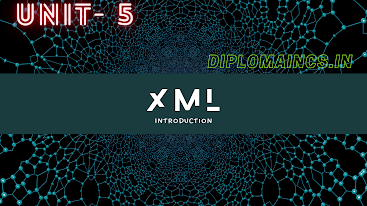## 12/28/20

### Computer Organization and Architecture question

Computer Organization and Architecture(Asked question for lateral entry)

Sub Code: - 1618304

(Lateral Entry)

2019(ODD)

Time : 3Hrs

Semester  III(New)/CSE

COA

Full Marks : 70

Pass marks : 28

Group A

Choose the most suitable answer from the following options: -   (1*20=20)

(i) A common bus system can be constructed using.

(a) Decoders

(b) Multiplexers

(c)  Registers

(d) Decoders and multiplexers

(ii) The process by which the requesting processor may be granted the access to the bus is known as: -

(a) Bus granting

(b) Bus arbitration

(c) Bus sharing

(d) Bus distribution

(iii) In signed-magnitude binary division, if the dividend is (11100)2 and divisor is (10011)2 then result is,

(a) (00100)2

(b) (10100)2

(c) (11001)2

(d) (01100)2

(iv) In computers, subtraction is carried out generally by

(a) 1’s complement method

(b) 2’s complement method

(c) Signed magnitude method

(d) BCD subtraction method

(v) In a computer with 16-bit word the largest signed numbers is

(a) 216

(b) 216-1 + 1

(c) 216 - 1

(d)216 + 1

(vi) If a number represented is signed 2’s complement forms is 11011, then the decimal equivalent is

(a) (-13)10

(b) (-12)10

(c) (-19)10

(d) (51)10

(vii) The operation executed on data stored in registers is called:

(a) Macro operation

(b) Micro operation

(c) Bit operation

(d) Byte operation

(viii) A stack organized computers uses instruction of.

(ix) When CPU is executing a program that is a part of operating system, it is said to be in………………..

(a) Interrupt mode

(b) System mode

(c) Half mode

(d) Simplex mode

(x) ……………. register keeps track of the instructions stored in program stored in memory.

(b) Index register (XR)

(c) Program counter (PC)

(d) Accumulator (AC)

(xi) The addressing mode used in an instruction of the form ADD X, Y is…………

(a) Absolute

(b) Indirect

(c) Index

(d) None of these

(xii) A group of bits that tell the computers to perform a specific operation is known as

(a) Instruction code

(b) Micro-operation

(c) Accumulator

(d) Register

(xiii) Status bit is also known as:

(a) Binary bit

(b) Flag bit

(c) Signed bit

(d) Unsigned bit

(xiv) While performing operations the ALU takes data from the temporary memory storage area inside the CPU named:

(a)Floppy disk

(b) RAM

(c) Register

(d) CD

(xv) Cache memory acts between:

(a)  CPU and RAM

(b) RAM and ROM

(c)  CPU and hard disk

(d) None of these

(xvi) The average time required to reach a storage location in memory and obtain its content is-

(a)  Transfer time

(b) Access time

(c)  Seek time

(d) Response time

(xvii) An address in main memory is called:

(xviii) How many 128 x 8 RAM chips are needed to provide a memory capacity of 2048 bytes?

(a)  8

(b) 16

(c)  24

(d) 32

(xix) The communication between the components in a microcomputer take places via the address and

(a)  I/O bus

(b) Data bus

(c)  Interface

(d) Control lines

(xx) An interrupt for which hardware automatically transfer the program to a specific memory location is known as-

(a)  Software interrupts

(b) Hardware interrupts

(d) Vector interrupts

Group:-"B"

Answer all Five Questions: -                         (5*4=20)

2.  List various input output devices and discuss about them in brief.

OR

With the help of block diagram, discuss working of direct memory access?

3.  Compare memory mapped F/O v/s I/O

OR

With neat diagram, explain the strobe control data transfer method.

4. What do you mean by virtual and physical address of memory? Explain with an example.

OR

Given the cache address access time as 10 ns.memory access time as 100ns  and cache rate as 90% calculate the effective memory access time.

5. How are direct access memories different from random access memories?

OR

What do you mean by instruction format?

6. How many bits are needed to represent an instruction in a computer that has 53 different instructions, all of which are two addresses, when the computer has 65000 word memories?

OR

Group:- "C"

Answer all Five Questions: -                         (5*6=30)

7.  What is an instruction? What are the different parts of an instruction? Explain the significant of each part of an instruction with an example?

OR

Explain with an example, how effective address is calculated if different types of addressing modes.

8.  Write short notes on the following:

i.          Micro instructions

ii.          Micro program

iii.          Control memory

OR

Explain the difference between a micro programmed control unit a hardware control unit.

9.  Explain Booth’s multiplications algorithm through an example.

OR

Discuss addition and subtraction algorithm with the help of flowchart.

10.  Perform the following conversion:

a.          (346)10 -> (?)2

b.          (76A)10­ -> (?)2

c.          (A5C)10  -> (?)2

OR

Multiply the following binary number:

a.    11012 x 1012

b.    101012 x 11002

c.     11002 x 10100012

11.  Describe the following organization of cache memory:

a.    Associative mapping

b.    Direct mapping

c.     Set – Associated mapping

OR

Explain the following in brief: